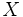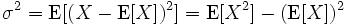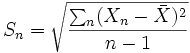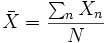# Standard deviationThis article/section deals with mathematical concepts appropriate for late high school or early college.

Standard deviation is a measure in statistics of the dispersion of a set of values (represented as). It is defined as the square root of the variance of these values, where variance is defined aswhere the expected value of X is E(X).

Thus the standard deviation isThe formula for standard deviation must not be confused with the formula(whereis the sample mean).

which is the formula for a point estimate of the true standard deviation from a sample size of n. As such this statistical estimator itself has a variance which, as the formula indicates, decreases as the sample size increases.